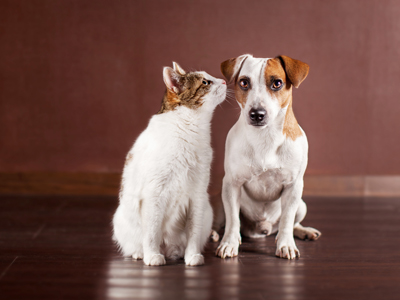What percentage of people prefer cats to dogs?

# Percentages 3 (Difficult)

So, you have made it to the third of our Difficult level Eleven Plus maths quizzes on Percentages – give yourselves a pat on the back! As you will know by now, percentages are a way to represent proportions of numbers, but how do you calculate them? In the last quiz we showed you one method. Here’s another which you might prefer:

What percentage of 70 is 28?
First divide 70 by 100 to work out what 1% of 70 is:
70 ÷ 100 = 0.7
Next, divide 28 by 0.7 to work out how many “lots” of 1% are in 28:
28 ÷ 0.7 = 40

This method is great for estimating your answers, but it often requires a calculator!

So now, on with the quiz. You can use the method above, or the one we showed you in our previous quiz. Alternatively, you may have your own method. Use whichever you prefer – as long as it gives you the right answer!

1.
What percentage of 72 is 48?
33.33%
60%
66.66%
70%
(48 ÷ 72) x 100 = (48 × 100) ÷ 72 = 4,800 ÷ 72 = 66.66%
If you know your times tables, you might have spotted that 72 is 6 x 12 and 48 is 4 x 12. 4 is two-thirds of 6 so the percentage will be equivalent to two-thirds, i.e. 66.66%
2.
What percentage of 400 is 60?
1.5%
12.5%
15%
17.5%
(60 ÷ 400) × 100 = (60 × 100) ÷ 400 = 15%
Of course, you may have spotted that 400 is 4 x 100, so you could work this out more quickly by just dividing 60 by 4: 60 ÷ 4 = 15
3.
1,500 people were asked whether they prefer dogs or cats. 720 said “dogs”, 660 said “cats” and 120 said “neither”. What percentage of those surveyed prefer cats?
44%
33%
55%
66%
We can ignore the people who prefer dogs, or who said “neither”. All we have to do is work out what percentage of 1,500 660 is:
(660 ÷ 1,500) x 100 = (660 x 100) ÷ 1,500 = 66,000 ÷ 1,500 = 44%
4.
If there are 320 cars in a car park, and 96 of them are red, what percentage of cars in the car park are not red?
30%
55%
65%
70%
(96 ÷ 320) x 100 = (96 × 100) ÷ 320 = 9,600 ÷ 320 = 30%
That is the amount of red cars, but we want to know the percentage that are not red:
100 - 30 = 70, so 70% of the cars are not red
5.
What percentage of 8 is 30?
27%
375%
30%
350%
(30 ÷ 8) x 100% = (30 × 100) ÷ 8 = 3,000 ÷ 8 = 375%
In ‘top-heavy’ fractions (e.g. 308), the percentage will be more than 100%
6.
What percentage of 240 is 12?
2.5%
5%
10%
15%
(12 ÷ 240) x 100 = (12 × 100) ÷ 240 = 1,200 ÷ 240 = 5%
7.
A charity raffle sold 800 tickets for £1 each, making £800. They gave away £72 in prizes. What percentage of the money they raised went on prizes?
15%
13%
11%
9%
We are trying to find what percentage of 800 72 is:
(72 ÷ 800) × 100 = (72 × 100) ÷ 800 = 7,200 ÷ 800 = 9%
8.
A survey asked 8,000 people in the UK which religion they followed. 1,840 said that they had no religion. What percentage does this represent?
19%
21%
23%
25%
(1,840 ÷ 8,000) x 100 = (1,840 × 100) ÷ 8,000 = 184,000 ÷ 8,000 = 23%
Don’t be put off by large numbers – they can often be rounded down by removing any zeros, e.g. 184,000 ÷ 8,000 is the same as 184 ÷ 8
9.
What percentage of 900 is 81?
15%
13%
11%
9%
(81 ÷ 900) x 100 = (81 × 100) ÷ 900 = 8,100 ÷ 900 = 9%
Of course, 900 is 100 x 9, so you could work the answer out by simply dividing 81 by 9:
81 ÷ 9 = 9
10.
If 20% of us are vegetarian, how many vegetarians will there be in a randomly chosen group of 40 people (approximately)?
8
10
12
16
This question asks you to reverse the usual process:
To find 20% of 40, divide by 100 then times by 20:
40 ÷ 100 = 0.4
0.4 x 20 = 8
A quicker way to find 20% is to simply divide by 5 (because 20% is the same as one-fifth)
40 ÷ 5 = 8
Author:  Frank Evans